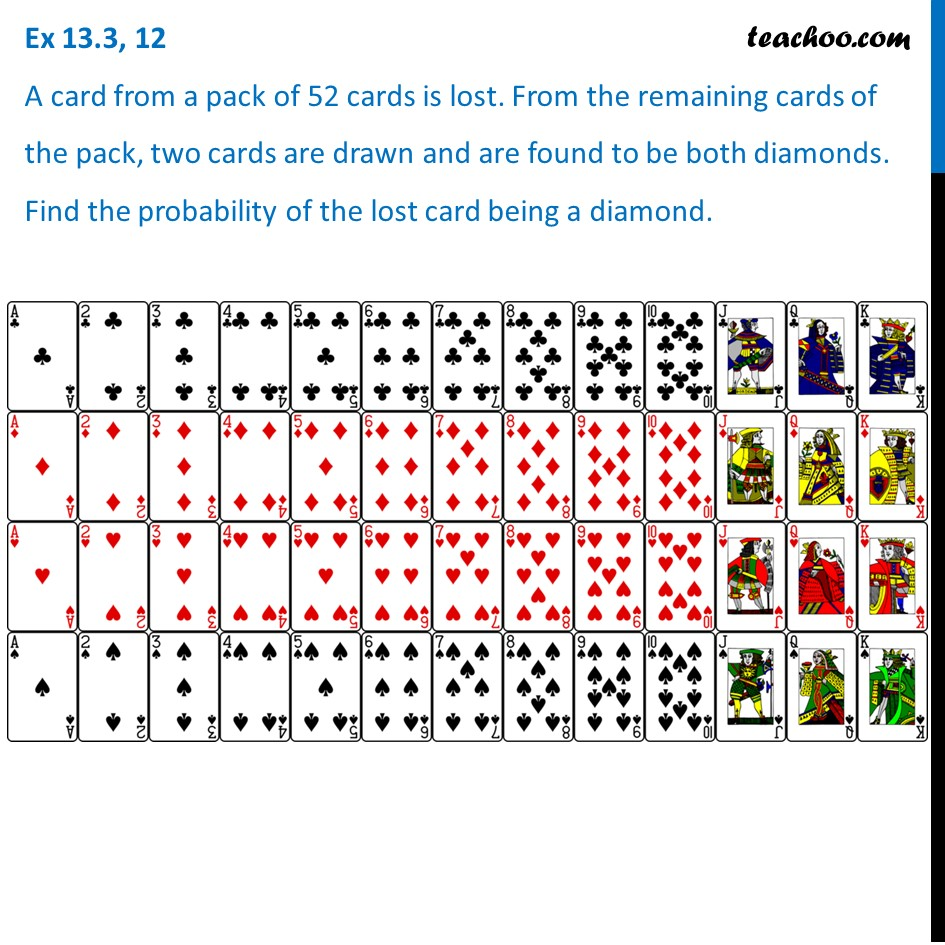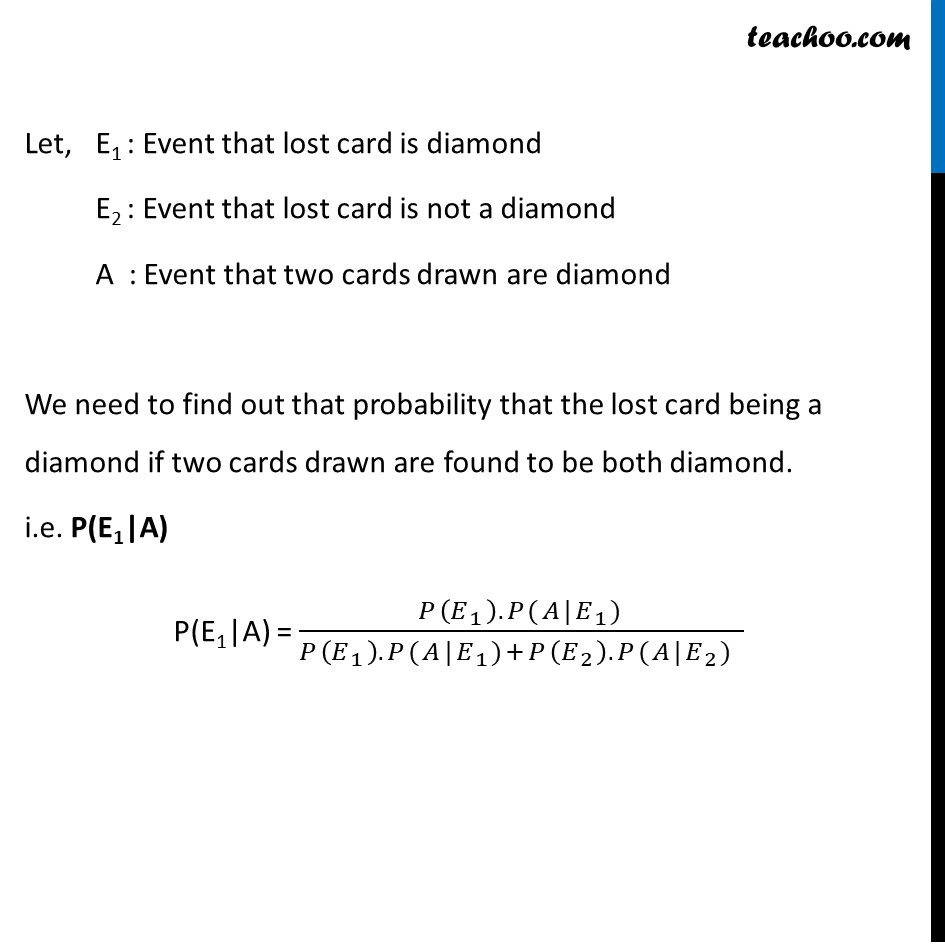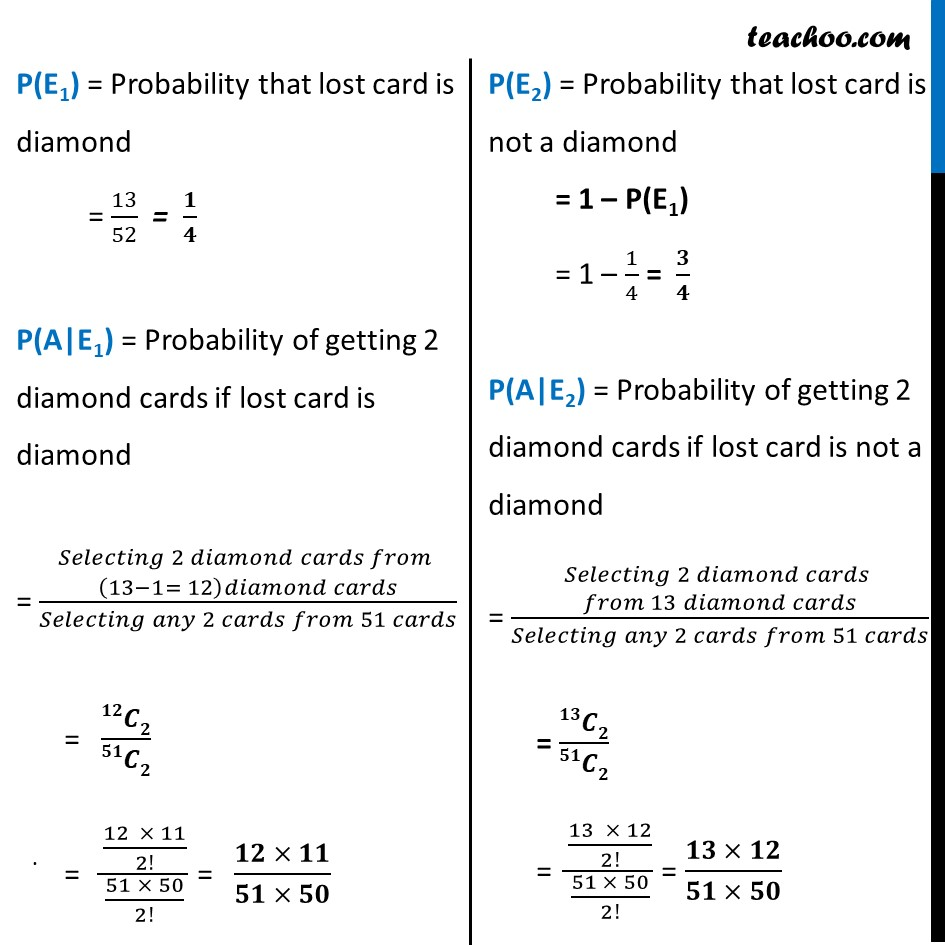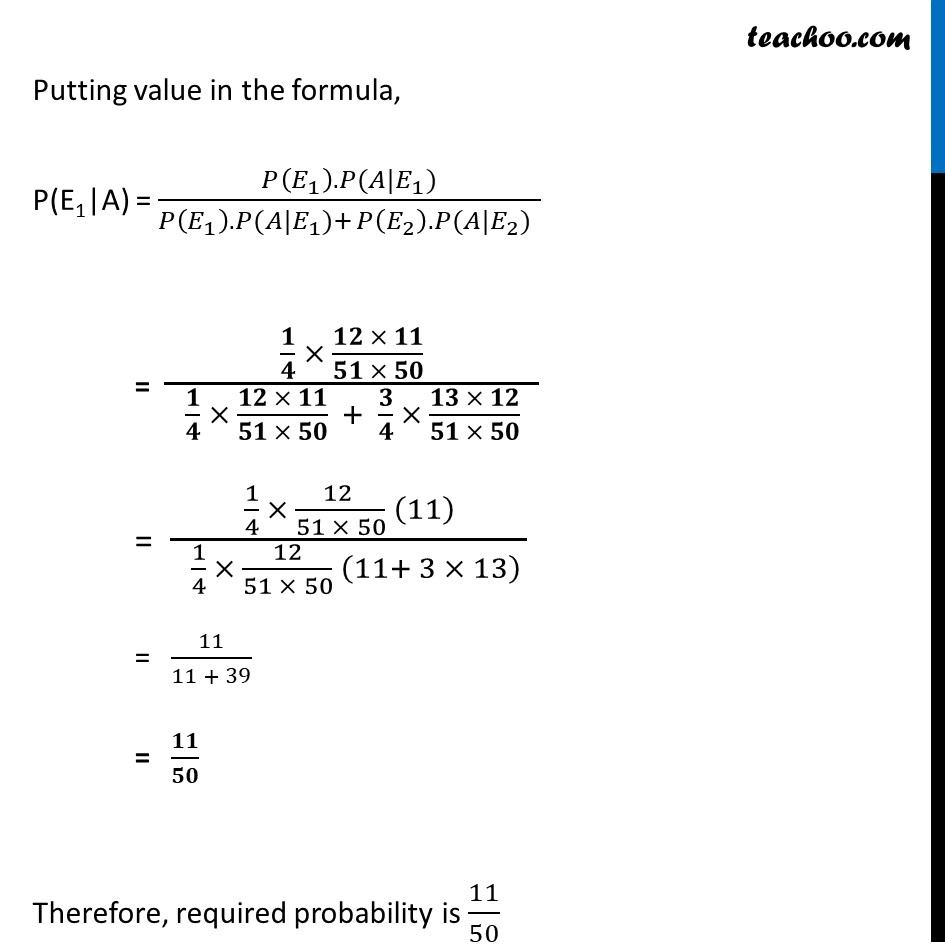Ex 13.3

Chapter 13 Class 12 Probability
Serial order wiseLearn in your speed, with individual attention - Teachoo Maths 1-on-1 Class

### Transcript

Ex 13.3, 12 A card from a pack of 52 cards is lost. From the remaining cards of the pack, two cards are drawn and are found to be both diamonds. Find the probability of the lost card being a diamond.Let, E1 : Event that lost card is diamond E2 : Event that lost card is not a diamond A : Event that two cards drawn are diamond We need to find out that probability that the lost card being a diamond if two cards drawn are found to be both diamond. i.e. P(E1|A) P(E1|A) = (𝑃(𝐸_1 ).𝑃(𝐴|𝐸_1))/(𝑃(𝐸_1 ).𝑃(𝐴|𝐸_1)+𝑃(𝐸_2 ).𝑃(𝐴|𝐸_2) ) "P(E1)" = Probability that lost card is diamond = 13/52 = 𝟏/𝟒 P(A|E1) = Probability of getting 2 diamond cards if lost card is diamond = █(𝑆𝑒𝑙𝑒𝑐𝑡𝑖𝑛𝑔 2 𝑑𝑖𝑎𝑚𝑜𝑛𝑑 𝑐𝑎𝑟𝑑𝑠 𝑓𝑟𝑜𝑚 @(13−1= 12)𝑑𝑖𝑎𝑚𝑜𝑛𝑑 𝑐𝑎𝑟𝑑𝑠)/(𝑆𝑒𝑙𝑒𝑐𝑡𝑖𝑛𝑔 𝑎𝑛𝑦 2 𝑐𝑎𝑟𝑑𝑠 𝑓𝑟𝑜𝑚 51 𝑐𝑎𝑟𝑑𝑠) = 𝟏𝟐𝑪𝟐/𝟓𝟏𝑪𝟐 = ((12 × 11)/2!)/((51 × 50)/2!) = (𝟏𝟐 × 𝟏𝟏)/(𝟓𝟏 × 𝟓𝟎) "P(E2)" = Probability that lost card is not a diamond = 1 – P(E1) = 1 – 1/4 = 𝟑/𝟒 P(A|E2) = Probability of getting 2 diamond cards if lost card is not a diamond = █(𝑆𝑒𝑙𝑒𝑐𝑡𝑖𝑛𝑔 2 𝑑𝑖𝑎𝑚𝑜𝑛𝑑 𝑐𝑎𝑟𝑑𝑠 @𝑓𝑟𝑜𝑚 13 𝑑𝑖𝑎𝑚𝑜𝑛𝑑 𝑐𝑎𝑟𝑑𝑠)/(𝑆𝑒𝑙𝑒𝑐𝑡𝑖𝑛𝑔 𝑎𝑛𝑦 2 𝑐𝑎𝑟𝑑𝑠 𝑓𝑟𝑜𝑚 51 𝑐𝑎𝑟𝑑𝑠) = 𝟏𝟑𝑪𝟐/𝟓𝟏𝑪𝟐 = ((13 × 12)/2!)/((51 × 50)/2!) = (𝟏𝟑 × 𝟏𝟐)/(𝟓𝟏 × 𝟓𝟎) Putting value in the formula, P(E1|A) = (𝑃(𝐸_1 ).𝑃(𝐴|𝐸_1))/(𝑃(𝐸_1 ).𝑃(𝐴|𝐸_1)+𝑃(𝐸_2 ).𝑃(𝐴|𝐸_2) ) = (𝟏/𝟒 × (𝟏𝟐 × 𝟏𝟏)/(𝟓𝟏 × 𝟓𝟎))/( 𝟏/𝟒 × (𝟏𝟐 × 𝟏𝟏)/(𝟓𝟏 × 𝟓𝟎) + 𝟑/𝟒 × (𝟏𝟑 × 𝟏𝟐)/(𝟓𝟏 × 𝟓𝟎) ) = (1/4 × (12 )/(51 × 50) (11))/( 1/4 × 12/(51 × 50) (11+ 3 × 13) ) = 11/(11 + 39) = 𝟏𝟏/𝟓𝟎 Therefore, required probability is 11/50